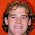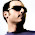## Sunday, February 8, 2009

### Derivation of the Navier Stokes and Fluid Transport Equations

In this series:
1. The Material Derivative
2. The Reynolds Transport Theorem
3. The Continuity Equation
4. The Momentum Equations
1. How Euler Derived the Momentum Equations
2. Cartesian Coordinates
3. Cylindrical Coordinates

Cite as:
Saad, T. "Derivation of the Navier Stokes and Fluid Transport Equations". Weblog entry from Please Make A Note. https://pleasemakeanote.blogspot.com/2008/08/derivation-of-navier-stokes-equations_16.html

1.You are going to have enough material for a book when you are done with these posts.

2.I found the derivation of the substantive derivative in cylindrical coordinates extremely helpful. (Why are those derivations omitted from the texts? ) Too bad the post for the momentum equations in cylindrical c.s. has not been created yet.

Here is what I am struggling with. Consider a case of Newtonian, incompressible fluid. The momentum equations for the radial and angular directions have two additional viscosity terms. Where do they come from and what is their physical significance?

3.Hi Anton,

Thank you for your feedback. I am very glad to hear that these posts have been of help to you.

The question you raised is a very interesting one and I was going to discuss it in the posts for the momentum equations. Unfortunately, I haven't had a chance to do so as I am extremely busy at the current time.

However, to quickly address your question, please consider the following argument.

As you may already know, the viscous terms stem from the surface forces on a fluid element which are described by the divergence of the stress tensor. By expanding that in curvilinear coordinates, you will end up with derivatives of unit vectors (just like in the case of the material derivative). At the outset, you will recover some additional terms. I have worked out the math for that, and I will post it as soon as possible.

This is the mathematical reason of why these terms show up in curvilinear coordinates. Nonetheless, as far as I know, I don't think that they hold any specific physical significance. They are only a byproduct of the coordinate system that you have chosen so that the net viscous force on a fluid element will be the same regardless.

I hope that answers your question. If I find something else on the physicality of these terms, I will let you know.

4.I just wanted to say thanks. These posts really clarified my understanding of the fluid fundamentals, putting them in more accessible language.

5.Thanks man! Best description ever!! I will have to link to my blog once I get it more organized. I am working on one still for the past few weeks, and it just keeps growing! You know me I have to put as much information as possible, :P, hehe. It should be a nice post though. Why haven't we combined our post on the DES?! IS the DES actually dead?!

6.Thanks Tim! Always a pleasure to hear back from you. I don't know what's happening with DES, but we should probably copy our posts there as well.

7.hi
nice work

8.Thank you very much for the manuals, I'm gonna print it because it's a little big for read it here, thanks again.

9.The question you raised is a very interesting one and I was going to discuss it in the posts for the momentum equations. Unfortunately, I haven't had a chance to do so as I am extremely busy at the current time.

10.This is a perfect post there are some 'interesting things in these equations but I have to say that we need enough time to do it specially for me because I spend much time in the pharmacy.

11.Hello, this is a lot of useful info here. Thanks !
Have u derived the momentum equation in cylindrical co-ordinates ?

12.Thankz a lot for these derivations...
if possible pls upload the following derivations as well...in cartesian ,spherical and cylindrical coordinates...
1.energy
2.momentum
3.state

13.I don't know how to thank you enough for these. You, sir, are a saint among men.

14.plz post derivation of equation of motion in different coordinates.........:)